Examples

Chapter 1 Class 11 Sets (Term 1)
Serial order wise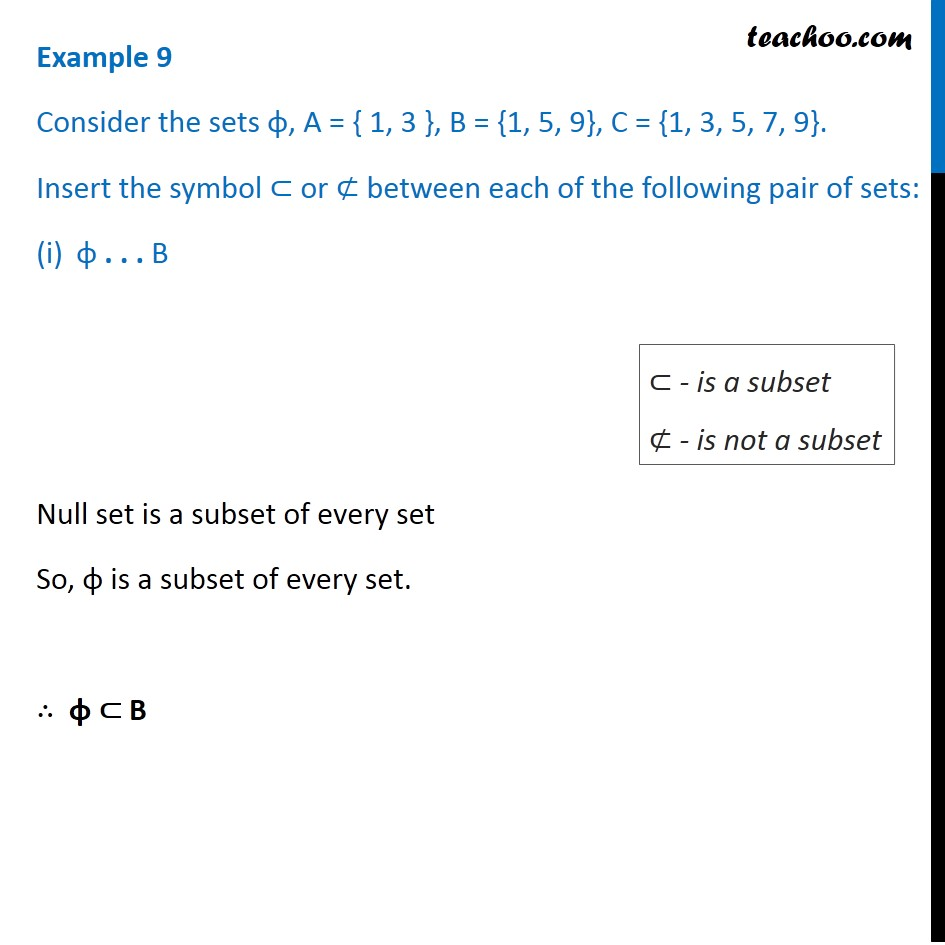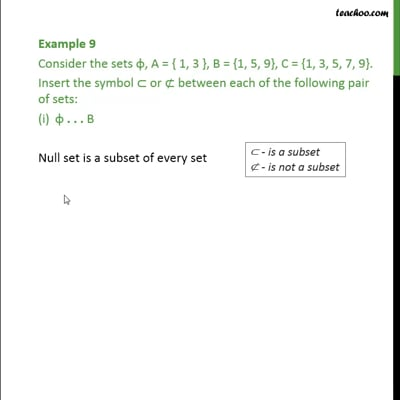This video is only available for Teachoo black users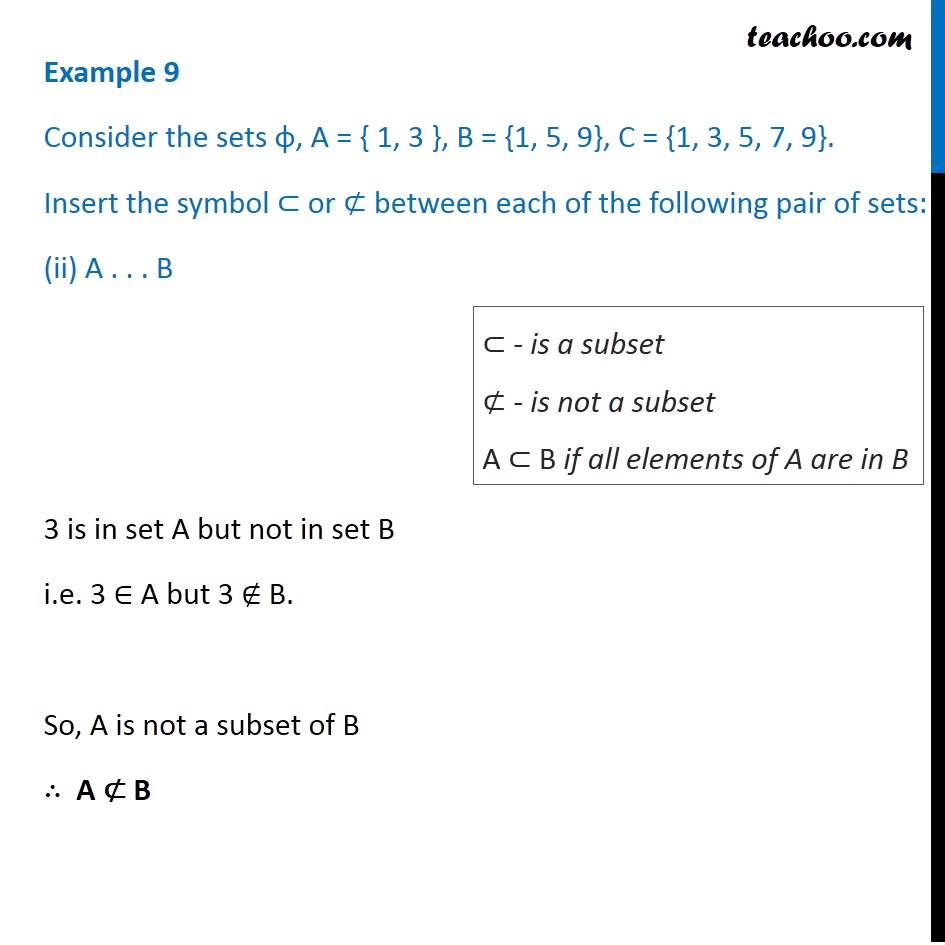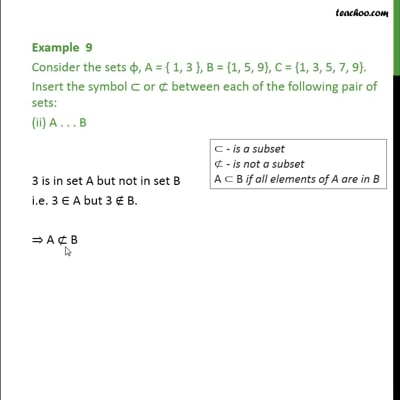This video is only available for Teachoo black users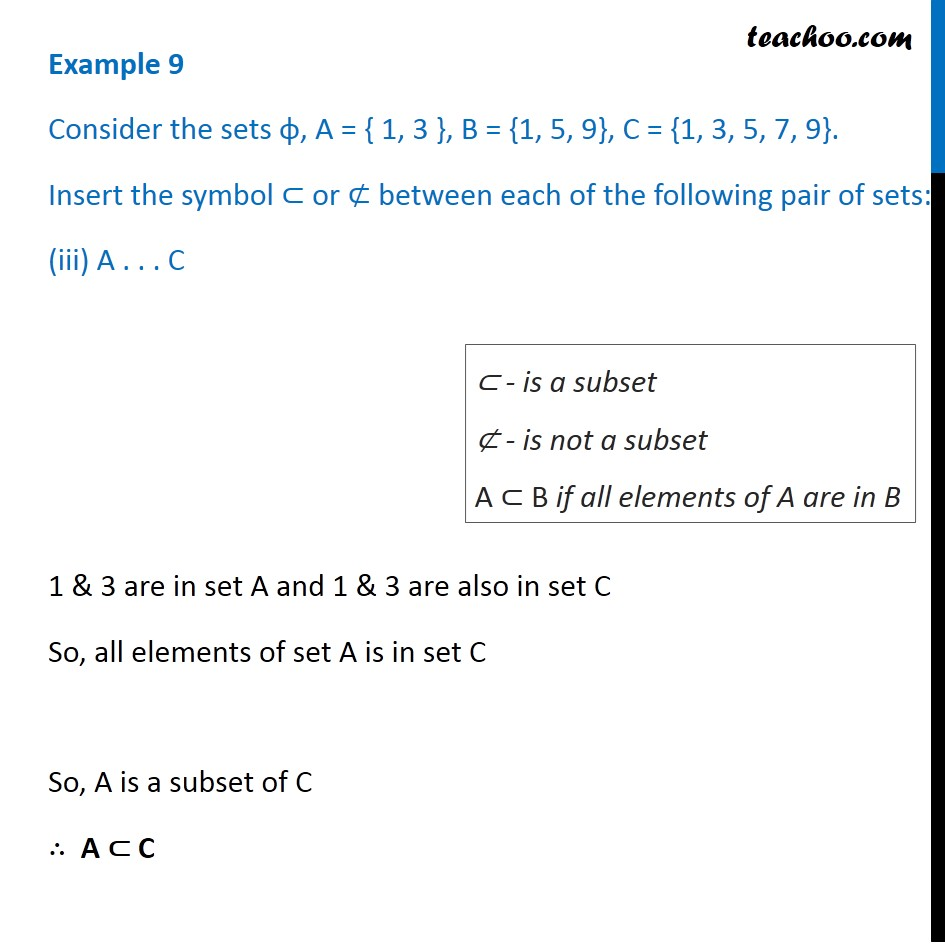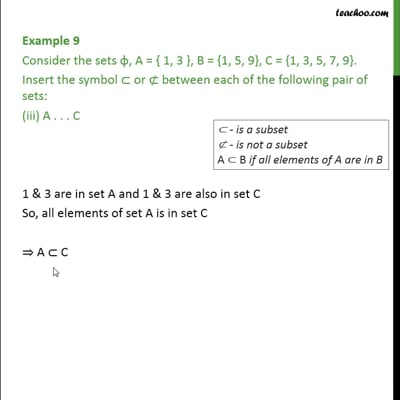This video is only available for Teachoo black users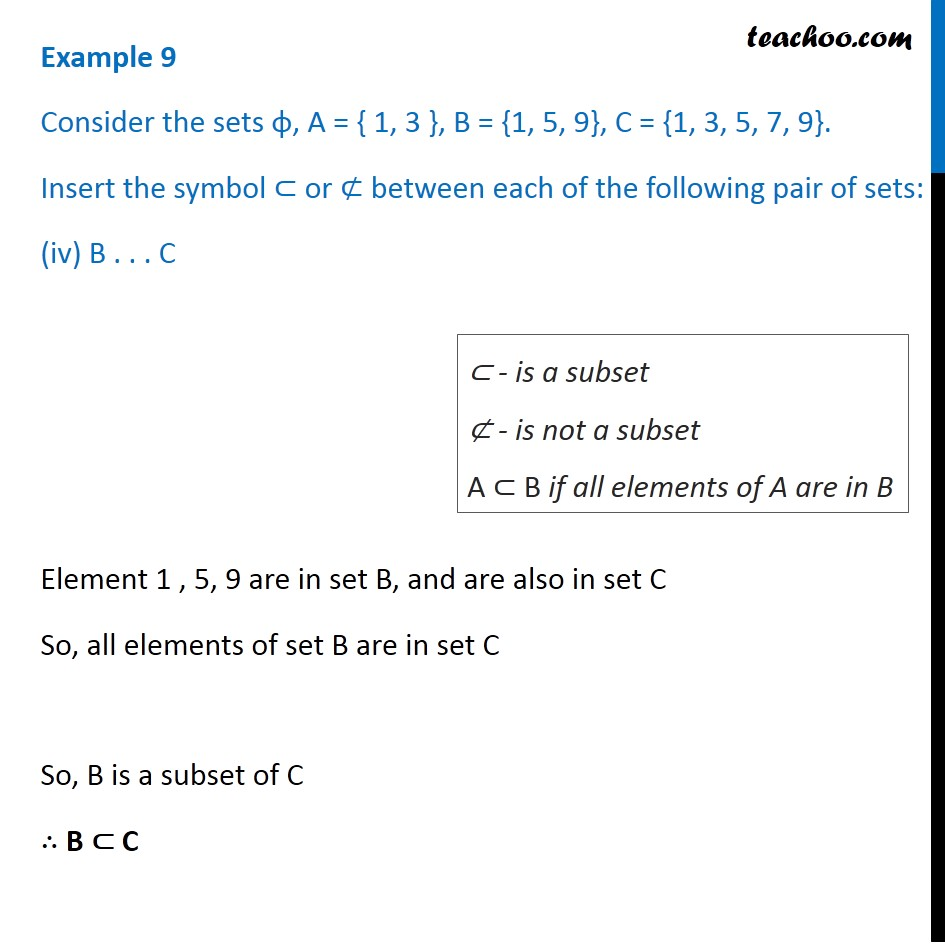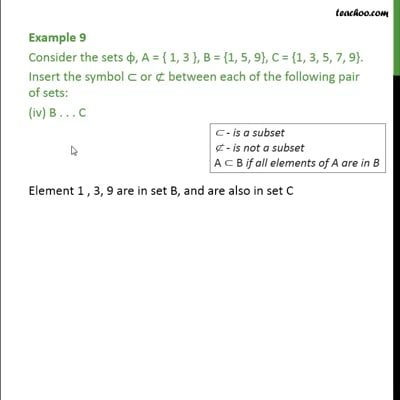This video is only available for Teachoo black users

### Transcript

Example 9 Consider the sets φ, A = { 1, 3 }, B = {1, 5, 9}, C = {1, 3, 5, 7, 9}. Insert the symbol ⊂ or ⊄ between each of the following pair of sets: φ . . . B Null set is a subset of every set So, φ is a subset of every set. ∴ φ ⊂ B ⊂ - is a subset ⊄ - is not a subset Example 9 Consider the sets φ, A = { 1, 3 }, B = {1, 5, 9}, C = {1, 3, 5, 7, 9}. Insert the symbol ⊂ or ⊄ between each of the following pair of sets: (ii) A . . . B 3 is in set A but not in set B i.e. 3 ∈ A but 3 ∉ B. So, A is not a subset of B ∴ A ⊄ B ⊂ - is a subset ⊄ - is not a subset A ⊂ B if all elements of A are in B Example 9 Consider the sets φ, A = { 1, 3 }, B = {1, 5, 9}, C = {1, 3, 5, 7, 9}. Insert the symbol ⊂ or ⊄ between each of the following pair of sets: (iii) A . . . C 1 & 3 are in set A and 1 & 3 are also in set C So, all elements of set A is in set C So, A is a subset of C ∴ A ⊂ C ⊂ - is a subset ⊄ - is not a subset A ⊂ B if all elements of A are in B Example 9 Consider the sets φ, A = { 1, 3 }, B = {1, 5, 9}, C = {1, 3, 5, 7, 9}. Insert the symbol ⊂ or ⊄ between each of the following pair of sets: (iv) B . . . C Element 1 , 5, 9 are in set B, and are also in set C So, all elements of set B are in set C So, B is a subset of C ∴ B ⊂ C ⊂ - is a subset ⊄ - is not a subset A ⊂ B if all elements of A are in B